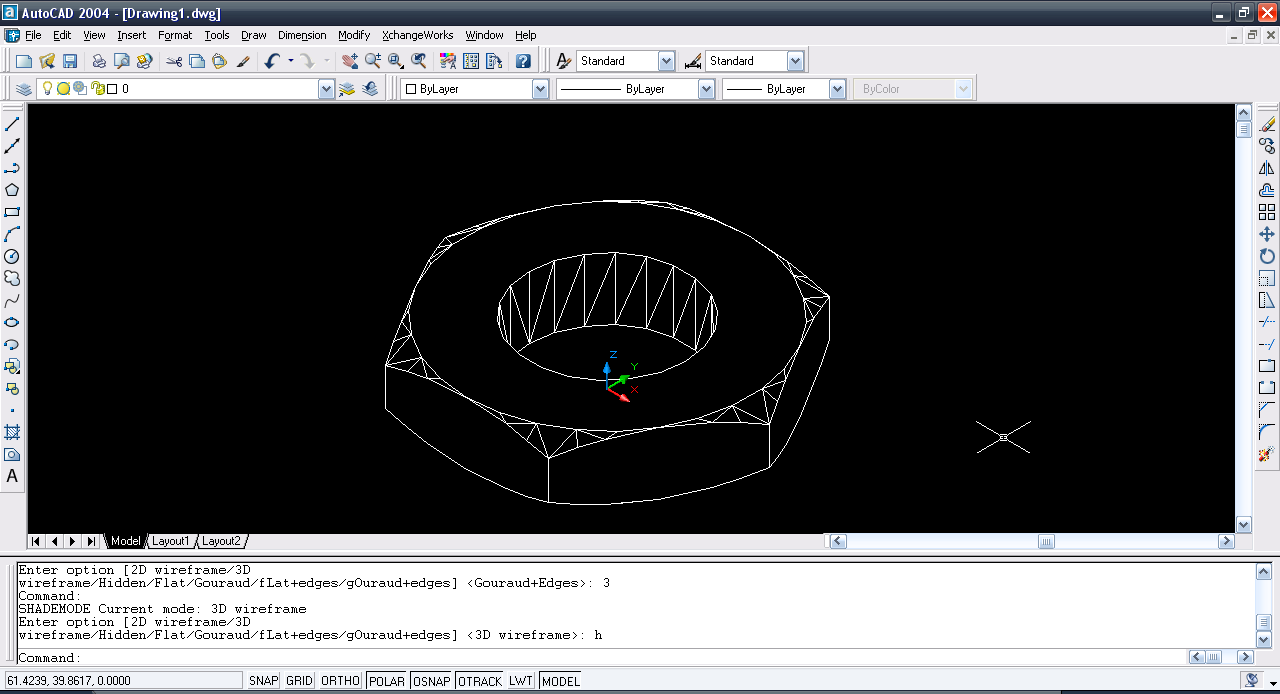# Tutorial - Making a Hex Nut in AutoCAD 2004?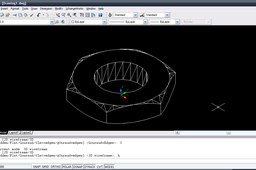Here goes the tutorial.

1. ### Step 1: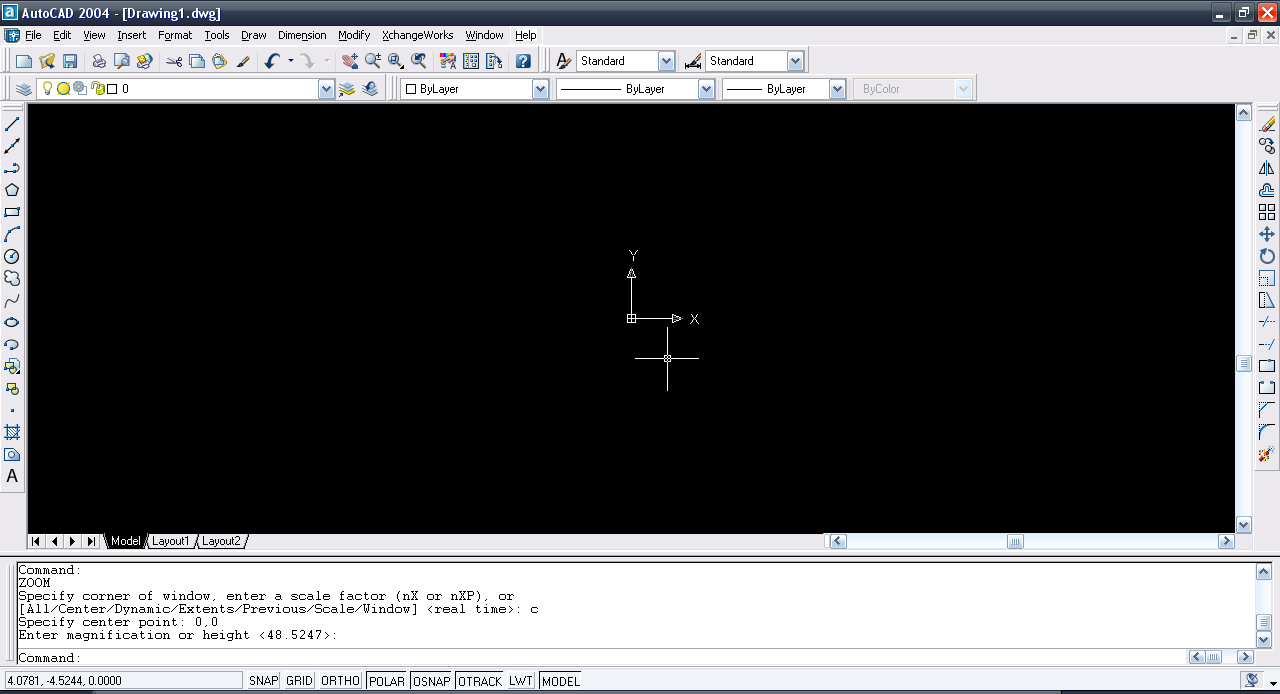2. ### Step 2:

Make a Polygon using polygon command with 6 side center at 0,0 circumscribed in a circle of radius 36mm.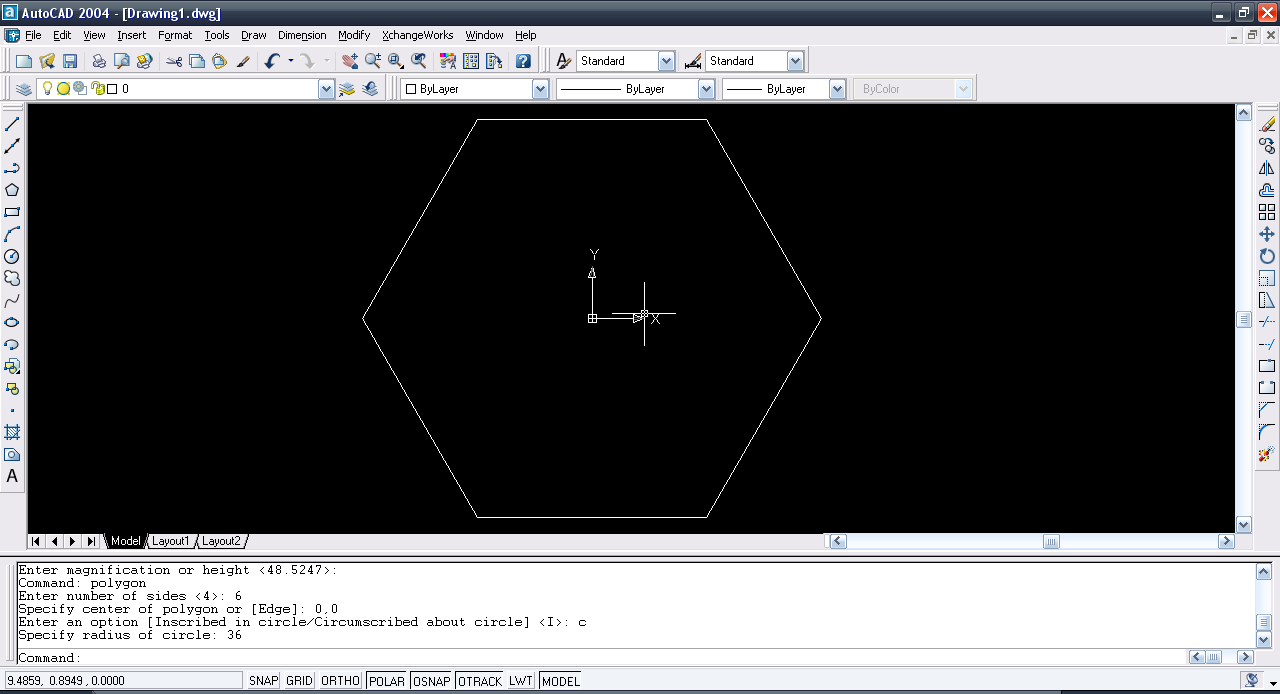3. ### Step 3:

Make a circle at origin of radius 20mm.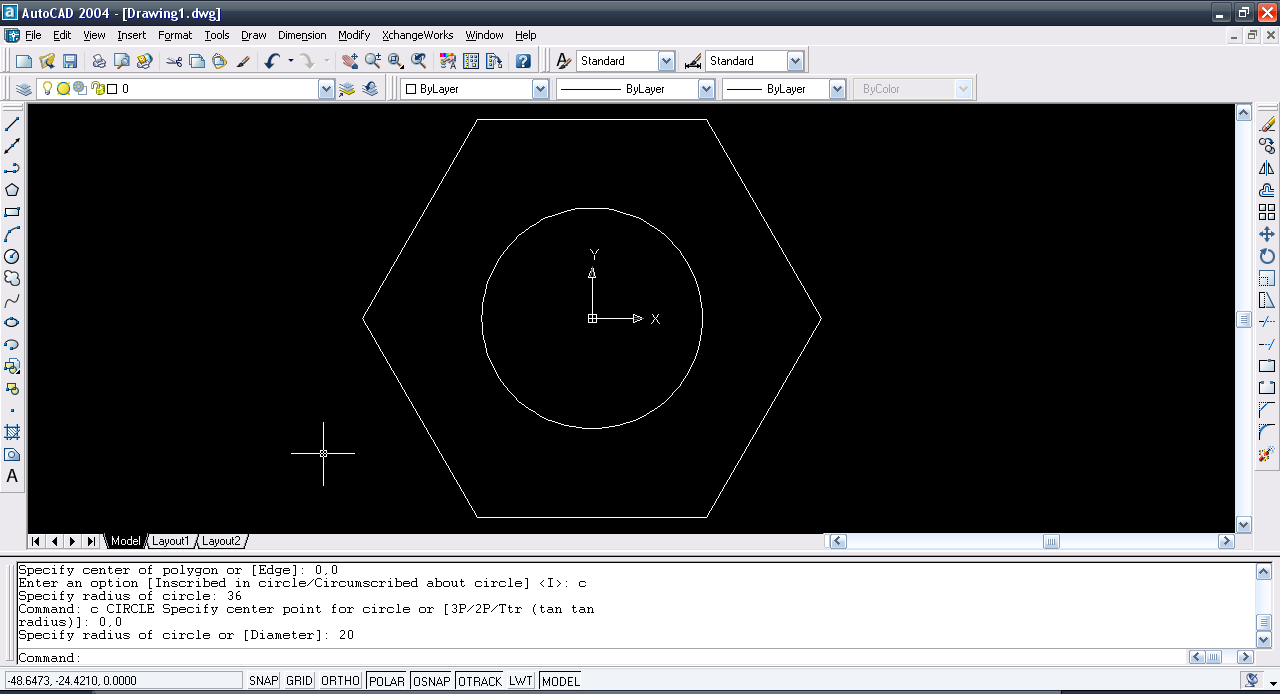4. ### Step 4:

Use region command and select all and then press enter. Then use Subtract Command and first select the polygon then enter and then select the circle and then again enter. Then Extrude it by a height of 8mm.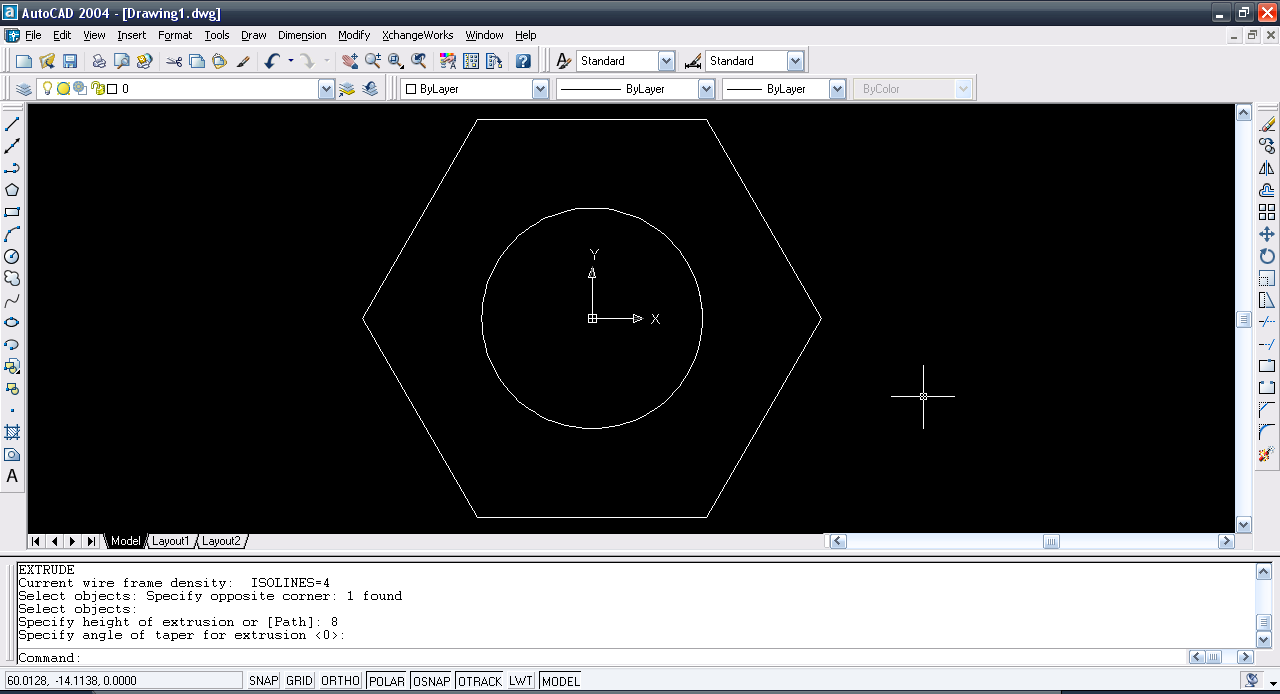5. ### Step 5:

Views>>3D Views>SE Isometric.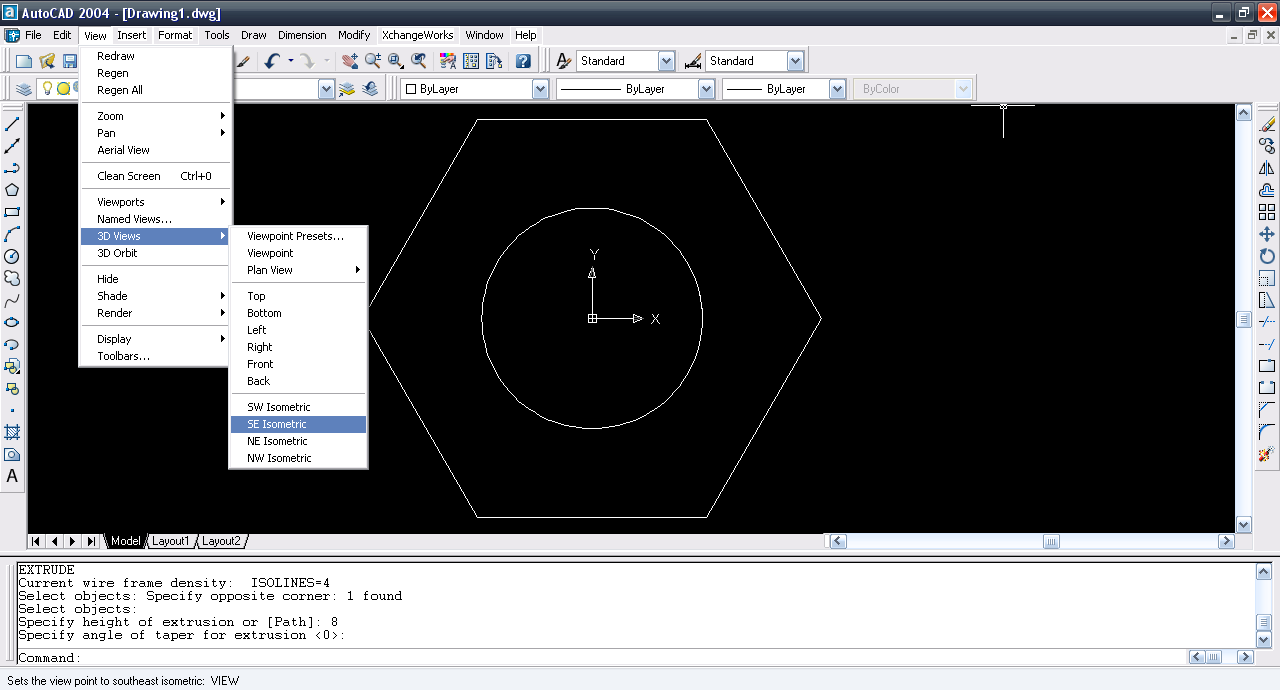6. ### Step 6:

Now make a circle with origin as center and tengent to the side of the polygon or simply of radius 36mm.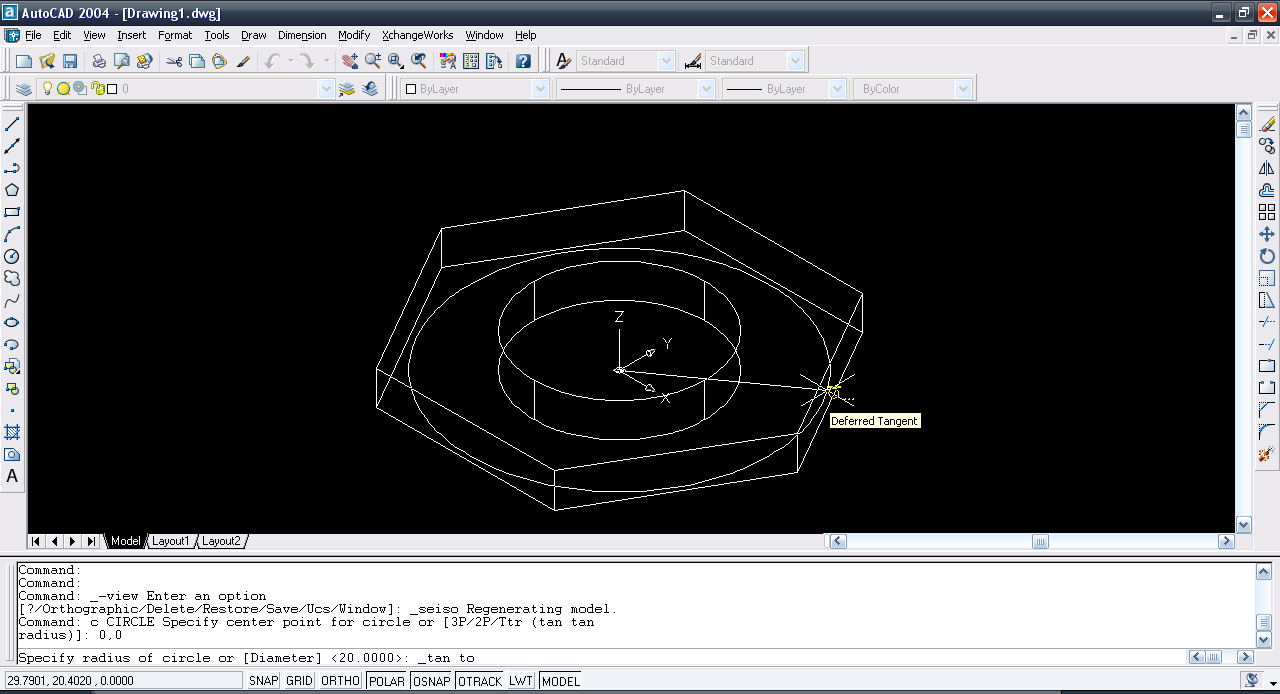7. ### Step 7:

Make a circle with origin as center but larger than previous one with a certain factor (say 60mm radius).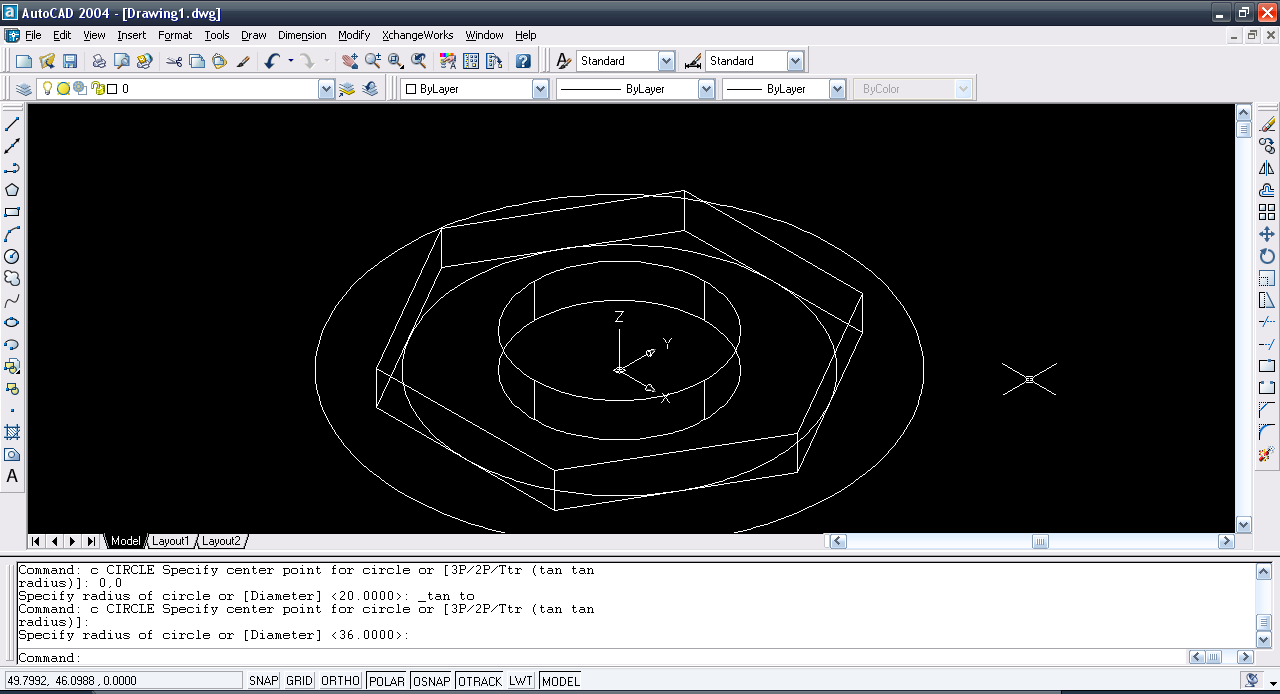8. ### Step 8:

Again Use region command and select all. Then Subtract Command and select larger circle first and then smaller circle and hit enter. Then Extrude the region left by a height of say 10mm with the taper angle of 60degrees.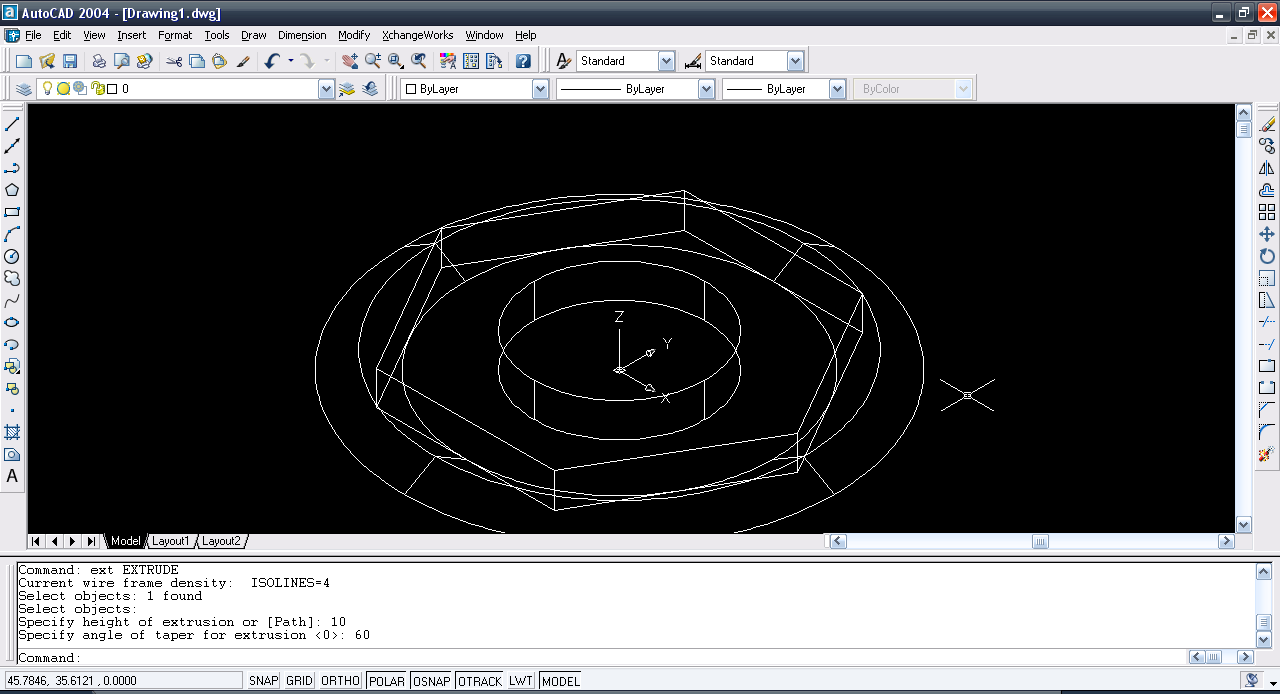9. ### Step 9:

Use subtract command and subtract the 2nd extruded body of the circles from the polygon and here is the first half of the hex nut.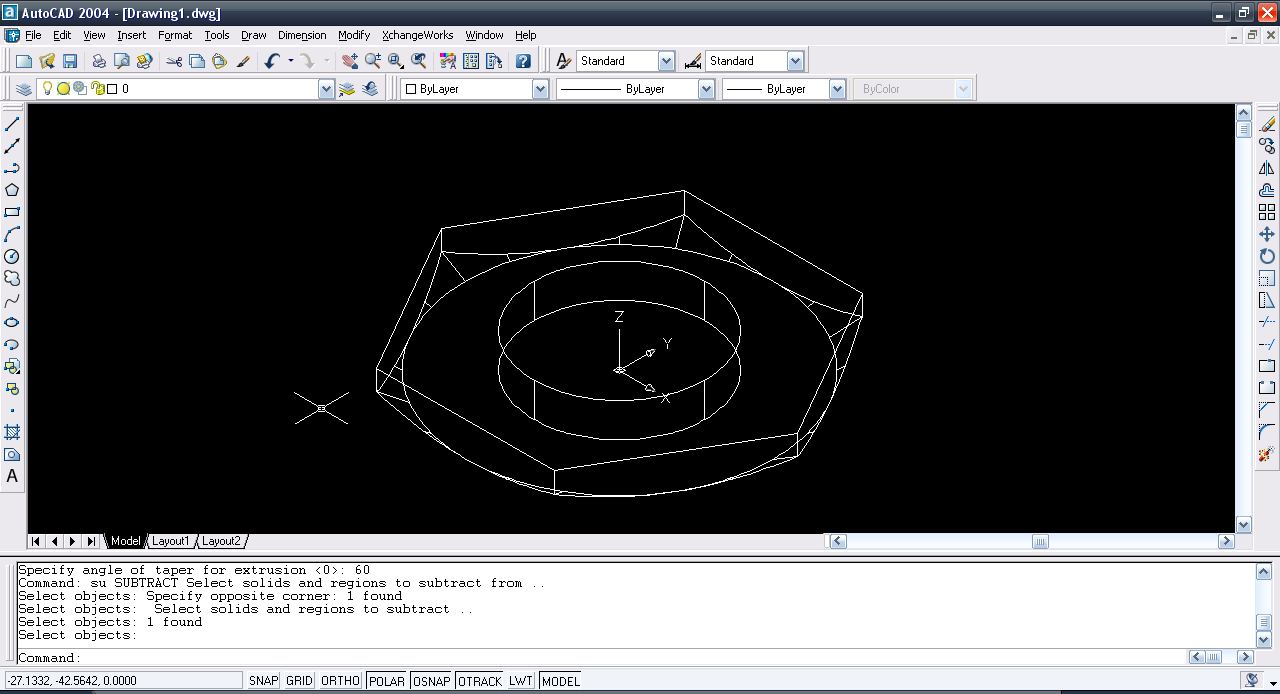10. ### Step 10: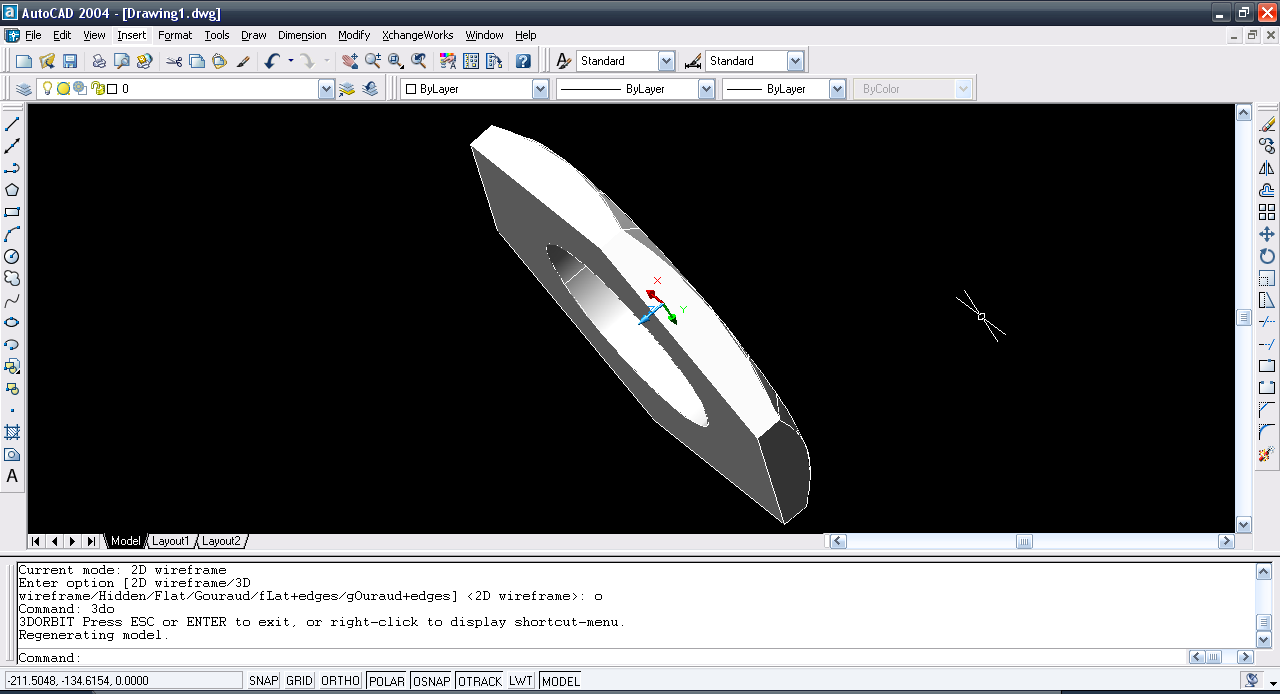11. ### Step 11:

use mirror3d command and select the polygon body and enter these points (0,0,8), (1,0,8), & (0,1,8). Say no to delete the original body.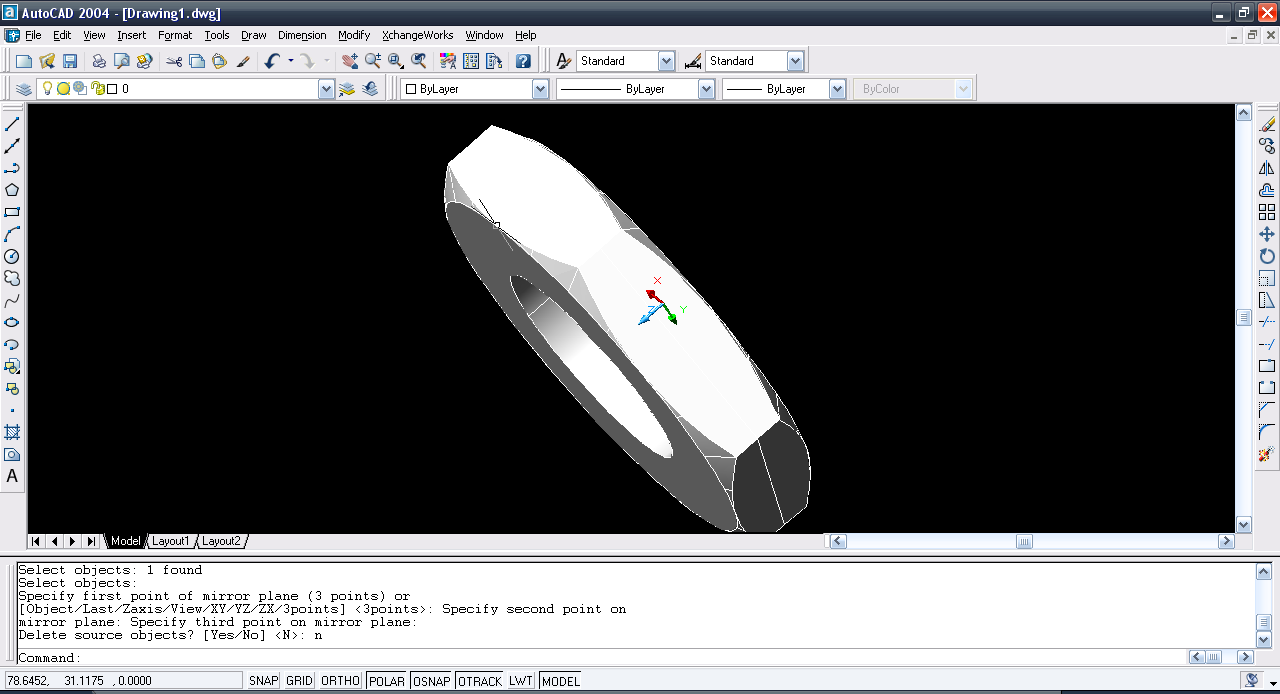12. ### Step 12:

Use union command and add them to form a single body. And here we have a Hex Nut.